ZHCSCB9E April   2014  – July 2022

PRODUCTION DATA

1. 特性
2. 应用
3. 说明
4. Revision History
5. Device Comparison Table
6. Pin Configuration and Functions
7. Specifications
8. Parameter Measurement Information
9. Detailed Description
10. 10Application and Implementation
1. 10.1 Application Information
2. 10.2 Typical Application
11. 11Power Supply Recommendations
12. 12Layout
13. 13Device and Documentation Support
14. 14Mechanical, Packaging, and Orderable Information

• DSG|8
• DSG|8

### 7.8.2 Typical Switching Characteristics

TA = 25°C, CT = 1000 pF, CIN = 1 µF, CL = 0.1 µF, RL = 10 Ω (unless otherwise specified). TA = 125°C data is only applicable to TPS22965NW-Q1 and TPS22965W-Q1.
TA = 25°C, CT = 1000 pF, CIN = 1 µF, CL = 0.1 µF, RL = 10 Ω (unless otherwise specified). TA = 125°C data is only applicable to TPS22965NW-Q1 and TPS22965W-Q1.VBIAS = 2.5 V CT = 1000 pF
Figure 7-14 tD vs VIN. TA = 25°C, CT = 1000 pF, CIN = 1 µF, CL = 0.1 µF, RL = 10 Ω (unless otherwise specified). TA = 125°C data is only applicable to TPS22965NW-Q1 and TPS22965W-Q1.VBIAS = 2.5 V CT = 1000 pF
Figure 7-16 tF vs VIN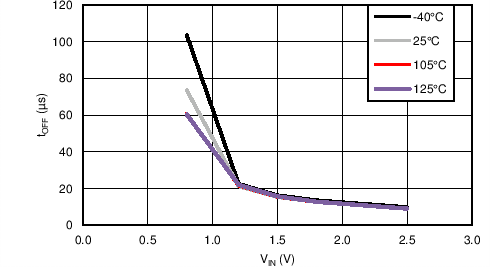VBIAS = 2.5 V CT = 1000 pF Note: The 105°C and 125°C curves have similar values; therefore, only one line is visible.
Figure 7-18 tOFF vs VINVBIAS = 2.5 V CT = 1000 pF
Figure 7-20 tON vs VINVBIAS = 2.5 V CT = 1000 pF Note: The 105°C and 125°C curves have similar values; therefore, only one line is visible.
Figure 7-22 tR vs VINVIN = 0.8 V VBIAS = 2.5 V CIN = 1 µF CL = 0.1 µF RL = 10 Ω CT = 1000 pF
Figure 7-24 Turn-On Response Time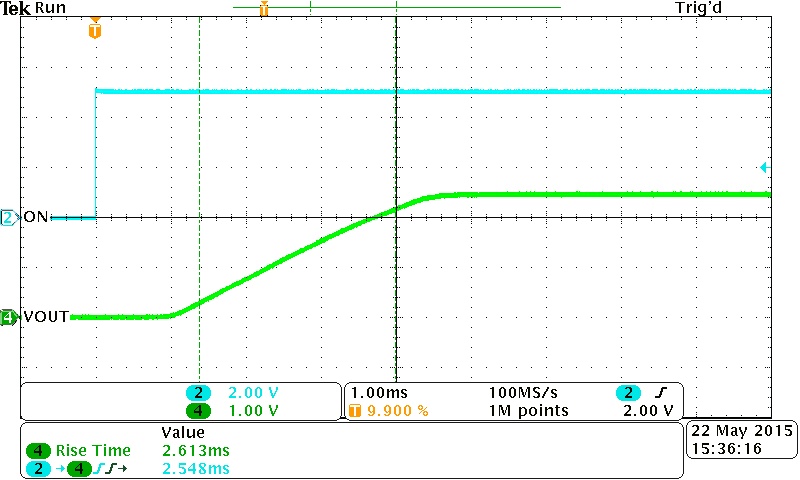VIN = 2.5 V VBIAS = 2.5 V CIN = 1 µF, CL = 0.1 µF RL = 10 Ω CT = 1000 pF
Figure 7-26 Turn-On Response TimeVIN = 0.8 V VBIAS = 2.5 V CIN = 1 µF CL = 0.1 µF RL = 10 Ω
Figure 7-28 Turn-Off Response TimeVIN = 2.5 V VBIAS = 2.5 V CIN = 1 µF CL = 0.1 µF RL = 10 Ω
Figure 7-30 Turn-Off Response TimeVBIAS = 5 V CT = 1000 pF
Figure 7-15 tD vs VIN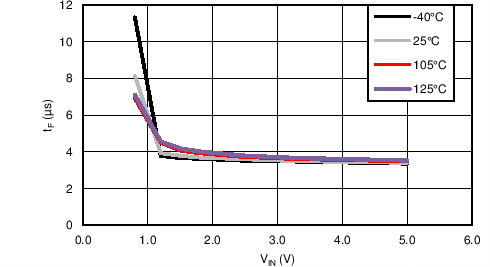VBIAS = 5 V CT = 1000 pF
Figure 7-17 tF vs VINVBIAS = 5 V CT = 1000 pF Note: The 105°C and 125°C curves have similar values; therefore, only one line is visible.
Figure 7-19 tOFF vs VIN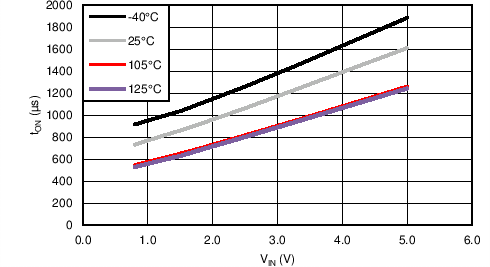VBIAS = 5 V CT = 1000 pF
Figure 7-21 tON vs VIN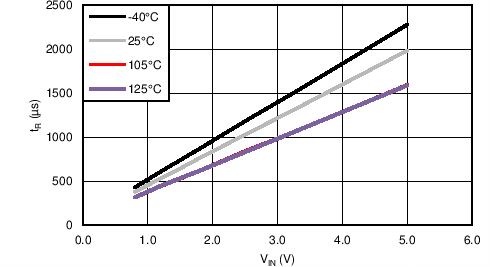VBIAS = 5 V CT = 1000 pF Note: The 105°C and 125°C curves have similar values; therefore, only one line is visible.
Figure 7-23 tR vs VIN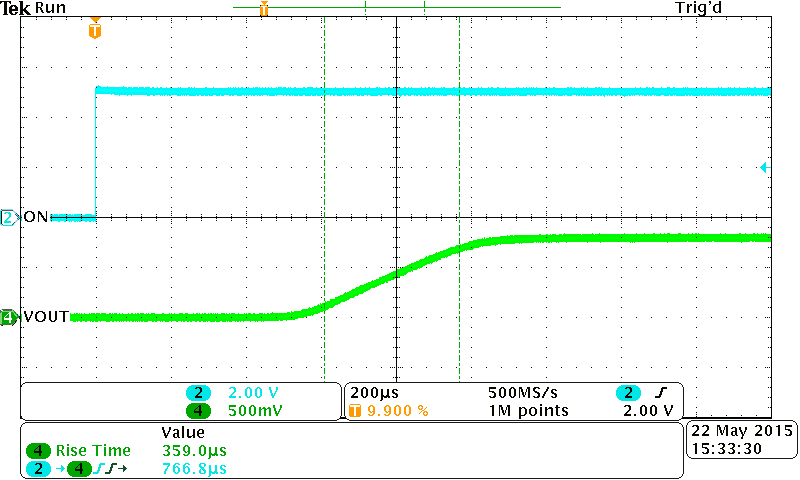VIN = 0.8 V VBIAS = 5 V CIN = 1 µF CL = 0.1 µF RL = 10 Ω CT = 1000 pF
Figure 7-25 Turn-On Response Time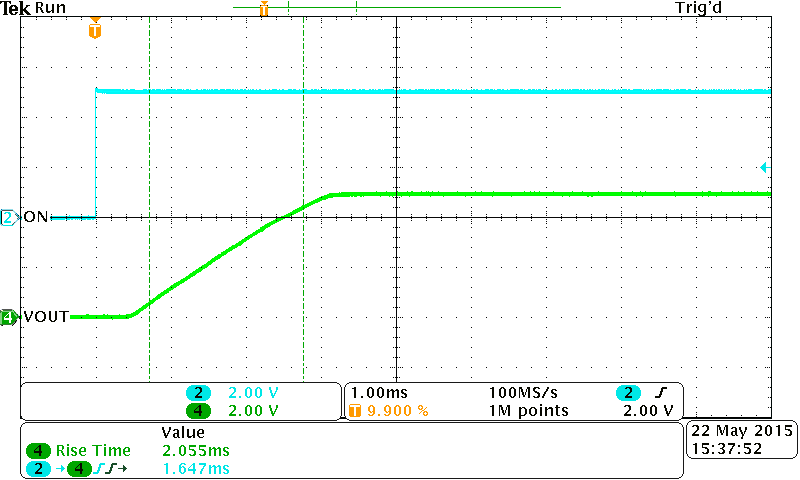VIN = 5 V VBIAS = 5 V CIN = 1 µF CL = 0.1 µF RL = 10 Ω
Figure 7-27 Turn-On Response TimeVIN = 0.8 V VBIAS = 5 V CIN = 1 µF CL = 0.1 µF RL = 10 Ω
Figure 7-29 Turn-Off Response TimeVIN = 5 V VBIAS = 5 V CIN = 1 µF CL = 0.1 µF RL = 10 Ω)
Figure 7-31 Turn-Off Response Time